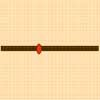# Resources tagged with: Turning points

Filter by: Content type:
Age range:
Challenge level:

### There are 12 results

Broad Topics > Calculus > Turning points### Exponential Trend

##### Age 16 to 18Challenge Level

Find all the turning points of y=x^{1/x} for x>0 and decide whether each is a maximum or minimum. Give a sketch of the graph.### Scientific Curves

##### Age 16 to 18Challenge Level

Can you sketch these difficult curves, which have uses in mathematical modelling?### Witch of Agnesi

##### Age 16 to 18Challenge Level

Sketch the members of the family of graphs given by y = a^3/(x^2+a^2) for a=1, 2 and 3.### Folium of Descartes

##### Age 16 to 18Challenge Level

Investigate the family of graphs given by the equation x^3+y^3=3axy for different values of the constant a.### Curve Fitter

##### Age 14 to 18Challenge Level

This problem challenges you to find cubic equations which satisfy different conditions.### Curve Hunter

##### Age 14 to 18Challenge Level

This problem challenges you to sketch curves with different properties.### Bird-brained

##### Age 16 to 18Challenge Level

How many eggs should a bird lay to maximise the number of chicks that will hatch? An introduction to optimisation.### Least of All

##### Age 16 to 18Challenge Level

A point moves on a line segment. A function depends on the position of the point. Where do you expect the point to be for a minimum of this function to occur.### Discrete Trends

##### Age 16 to 18Challenge Level

Find the maximum value of n to the power 1/n and prove that it is a maximum.### Quick Route

##### Age 16 to 18Challenge Level

What is the quickest route across a ploughed field when your speed around the edge is greater?### Patterns of Inflection

##### Age 16 to 18Challenge Level

Find the relationship between the locations of points of inflection, maxima and minima of functions.### Calculus Analogies

##### Age 16 to 18Challenge Level

Consider these analogies for helping to understand key concepts in calculus.